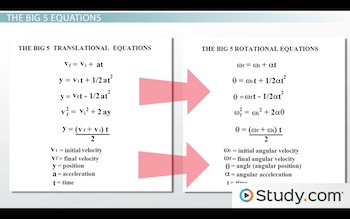# Five Kinematics Quantities & the Big 5 Equations

Lesson Transcript
Instructor: David Wood

David has taught Honors Physics, AP Physics, IB Physics and general science courses. He has a Masters in Education, and a Bachelors in Physics.

After watching this lesson, you will be able to list the five kinematics variables in rotational motion and use the five equations of constant acceleration to solve problems involving rotational motion. A short quiz will follow.

## Rotational Motion

A spinning top might not look very similar to a car racing down the highway. But when it comes to kinematics, they're not all that different. In another lesson, we discussed how every linear kinematic quantity has a rotational equivalent: instead of position, you have angle; instead of velocity, you have angular velocity; instead of acceleration, you have angular acceleration. And time is just. . . well, it's just time.

A spinning object operates in a very similar way. You have a certain velocity of rotation, which causes the angle (or angular position) to change over time, and that rotation velocity can increase or decrease, which is an angular acceleration. The relationships between these quantities are exactly the same as the relationships between the translational versions. Because of this, we were able to derive some basic kinematics equations for rotational motion just by taking a translational equation and replacing the variables with angular ones. Well, you can do the same thing for the 'big 5' equations of constant acceleration.An error occurred trying to load this video.

Try refreshing the page, or contact customer support.

Coming up next: Torque: Concept, Equation & Example

### You're on a roll. Keep up the good work!

Replay
Your next lesson will play in 10 seconds
• 0:03 Rotational Motion
• 1:12 The Big 5 Equations
• 2:55 Example Calculation
• 4:28 Lesson Summary
Save Save

Want to watch this again later?

Timeline
Autoplay
Autoplay
Speed Speed

## The Big 5 Equations

These are the 'big 5' kinematics equations - the five equations that describe a motion at a constant acceleration in a straight line. All we have to do to come up with the kinematic equations for rotational motion is replace each algebraic letter with the angular version. If we do that, the equations transform into these:In these equations, alpha is the angular acceleration measured in radians per second per second. Radians are just a measure of angle, like degrees, but whereas there are 360 degrees in a circle, there are 2-pi radians in the circle. Omega-I is the initial angular velocity, measured in radians per second. Omega-f is the final angular velocity, also measured in radians per second. Theta is the change in angle or angular position - so one full rotation would be a change in angle of 2-pi radians, for example. And t is the time it takes for the velocity to change from initial to final value. When solving problems with these new 'big 5' equations, we use the same strategies we've always used with the regular kinematics equations.

There are five variables and five equations. Each equation has a different combination of four of the five variables. In any given question, you will be given three numbers and asked to calculate a fourth. So, if you want to know which equation to use, go through them until you find the one that has the three givens you know and the one unknown you're trying to find. Then plug numbers in and solve. It's really that simple.

## Example Calculation

Okay, if it's really so simple, let's do an example. Let's say a tilt-a-whirl starts spinning from rest until it's spinning on its axis at its maximum speed of 10 radians per second. If the angular acceleration of the tilt-a-whirl is 2 radians per second per second, how long does it take to reach its maximum speed?

Well first of all, let's write out what we know. The initial angular velocity, omega-I, is zero because it starts from rest. The final angular velocity, omega-F, is 10 radians per second. And the angular acceleration, alpha, is 2 radians per second per second. We're asked to figure out t, the time it takes to reach that speed.

To unlock this lesson you must be a Study.com Member.

### Register to view this lesson

Are you a student or a teacher?